# Examples¶

We describe in this Section some examples of toy-problems that can be solved using Constraint Solving, and give the corresponding code in iZ-C.

## Image Decoding¶

Let us consider the following grid, where cells can be white or black: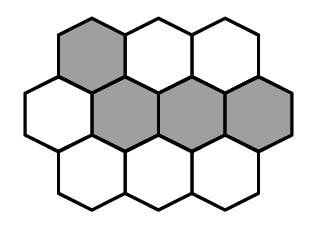This grid can be coded in writing in each cell the number of black neighbor cells (a cell is considered as a neighbor of itself).

For example, the coding for the grid above would be:The problem consists in finding the original image(s) whose coding is given below: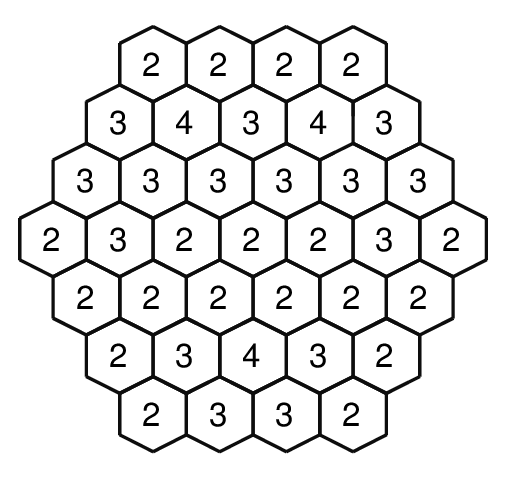Model:

Each cell of the grid will be described by a CSint variable whose possible values are 0 and 1. For each cell of the grid referenced by the index i, we will post a constraint:

cs_EQ(getSum(i, cell), Code[i]);


Which means that for a given cell referenced by i, the sum of the neighbor CSint variables must be equal to the Code[i].

getSum() is a user-defined function using the pre-defined cs_Sigma() constraint, returns the sum of the neighbors.

For the tree-search, we will use the pre-defined cs_findAll() function that search for all the solutions:

cs_findAll(cell, NB_CELLS, cs_findFreeVar, printSolution);


cs_findAll() is similar to cs_search() except that it takes as its fourth argument a function which will be called every time a solution is found.

iZ-C finds the four solution, and proves there is no more, within 9 backtracks. The four solutions are: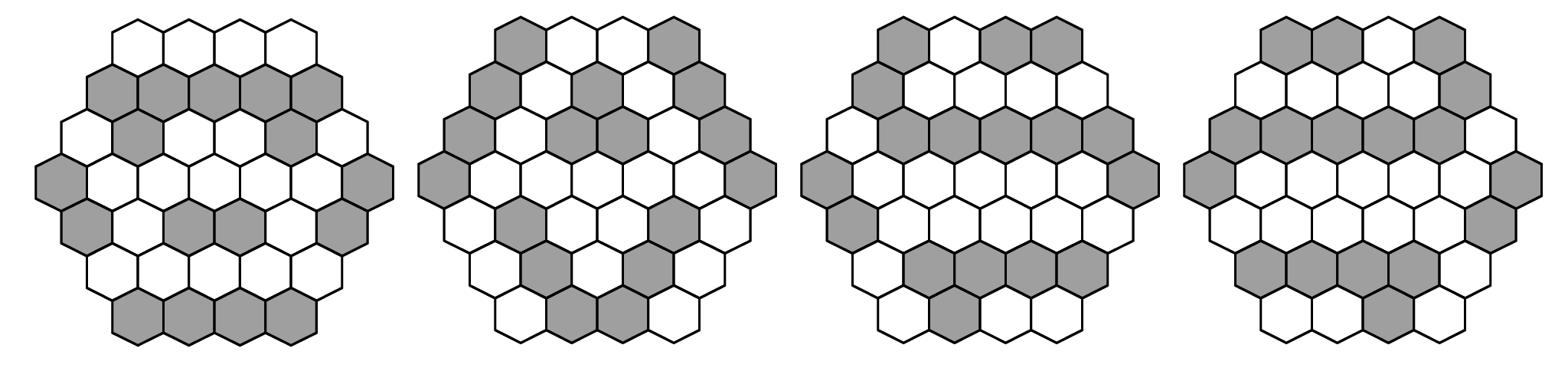The iZ-C code and the corresponding output is given below:

Code:

#include <stdlib.h>
#include <time.h>
#include "iz.h"

#define NB_CELLS 77
#define N -1

int Code[NB_CELLS] = {N,N,N,N,N,N,N,N,N,
N,N,2,2,2,2,N,N,
N,N,3,4,3,4,3,N,N,
N,3,3,3,3,3,3,N,
N,2,3,2,2,2,3,2,N,
N,2,2,2,2,2,2,N,
N,N,2,3,4,3,2,N,N,
N,N,2,3,3,2,N,N,
N,N,N,N,N,N,N,N,N};

void printCells(CSint **cell, int n, int nbCells)
{
int i;

for (i = 0; i < nbCells; i++) {
if (Code[i + n] != N)
printf("%d ", cs_getValue(cell[i + n]));
else
printf("  ");
}
printf("\n");
}

void printSolution(CSint **cell, int nbCells)
{
int i;
int n = 9;
static int NbSolutions = 0;

printf("\nSolution %d:\n", ++NbSolutions);
for (i = 1; i < 8; i++)
if (i % 2) {
printf(" ");
printCells(cell, n, 8);
n += 8;
}
else {
printCells(cell, n, 9);
n += 9;
}
}

CSint *getSum(int i, CSint **c)
{
CSint **array = (CSint**) malloc(7 * sizeof(CSint*));
int n = 0;

array[n++] = c[i];
if (Code[i + 1] != N) array[n++] = c[i + 1];
if (Code[i + 9] != N) array[n++] = c[i + 9];
if (Code[i + 8] != N) array[n++] = c[i + 8];
if (Code[i - 1] != N) array[n++] = c[i - 1];
if (Code[i - 9] != N) array[n++] = c[i - 9];
if (Code[i - 8] != N) array[n++] = c[i - 8];

return(cs_Sigma(array, n));
}

int main(int argc, char **argv)
{
int i;
clock_t t0 = clock();
CSint **cell;

cs_init();

cell = cs_createCSintArray(NB_CELLS, 0, 1);

for (i = 0; i < NB_CELLS; i++)
if (Code[i] == N)
cs_EQ(cell[i], 0);
else
cs_EQ(getSum(i, cell), Code[i]);

if (!cs_findAll(cell, NB_CELLS, cs_findFreeVar, printSolution))
printf("No solution\n");

cs_printStats();
printf("Elapsed Time = %fs\n", (double) (clock() - t0) / CLOCKS_PER_SEC);

cs_end();

return 0;
}


Output:

Solution 1:
0 0 0 0
1 1 1 1 1
0 1 0 0 1 0
1 0 0 0 0 0 1
1 0 1 1 0 1
0 0 0 0 0
1 1 1 1

Solution 2:
1 0 0 1
1 0 1 0 1
1 0 1 1 0 1
1 0 0 0 0 0 1
0 1 0 0 1 0
0 1 0 1 0
0 1 1 0

Solution 3:
1 0 1 1
1 0 0 0 0
0 1 1 1 1 1
1 0 0 0 0 0 1
1 0 0 0 0 0
0 1 1 1 1
0 1 0 0

Solution 4:
1 1 0 1
0 0 0 0 1
1 1 1 1 1 0
1 0 0 0 0 0 1
0 0 0 0 0 1
1 1 1 1 0
0 0 1 0

Nb Fails = 9
Nb Choice Points = 24
Heap Size = 1024
Elapsed Time = 0.000587s


It is interesting to note that for a given coding, there may be several corresponding solutions. If we change slightly the coding system (if the cell itself is now not considered as its neighbor), then one coding will give only one solution on a 37 cells grid.

## Multi Magic Square¶

The problem consists in finding the original image(s) when the sum of consecutive pixels are known .

Example :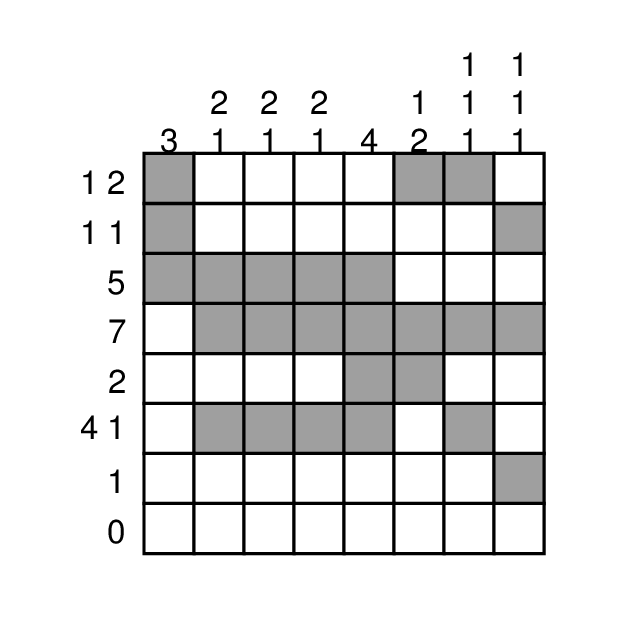There are in fact four solutions for this specific problem, found in 89 backtracks: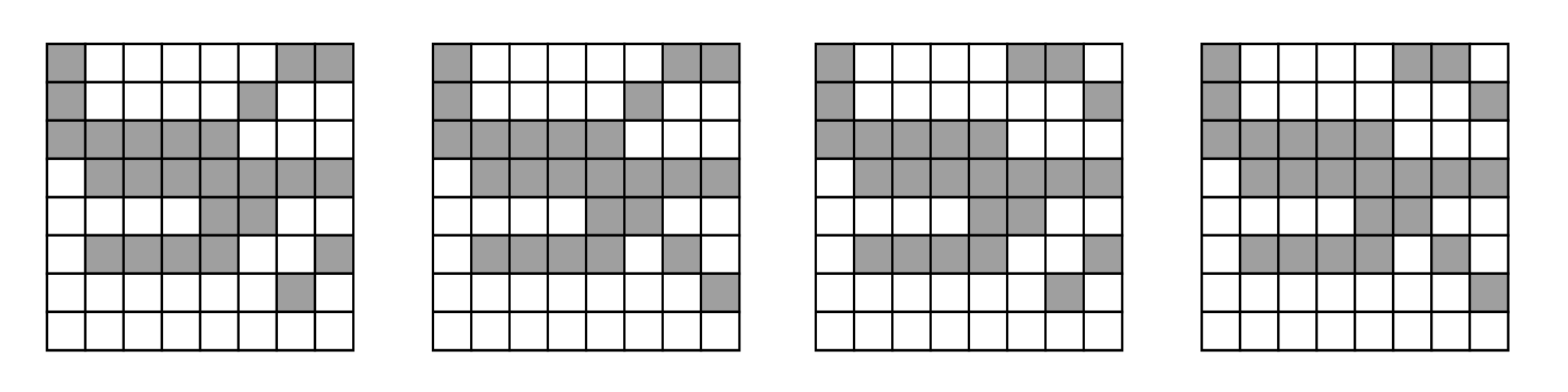The iZ-C code and the corresponding output is given below:

Code:

#include <stdlib.h>
#include <time.h>
#include "iz.h"

#define N 8

int row_1[] = {2, 1, 2};
int row_2[] = {2, 1, 1};
int row_3[] = {1, 5};
int row_4[] = {1, 7};
int row_5[] = {1, 2};
int row_6[] = {2, 4, 1};
int row_7[] = {1, 1};
int row_8[] = {1, 0};

int col_1[] = {1, 3};
int col_2[] = {2, 2, 1};
int col_3[] = {2, 2, 1};
int col_4[] = {2, 2, 1};
int col_5[] = {1, 4};
int col_6[] = {2, 1, 2};
int col_7[] = {3, 1, 1, 1};
int col_8[] = {3, 1, 1, 1};

int *row[N] = {row_1,
row_2,
row_3,
row_4,
row_5,
row_6,
row_7,
row_8};

int *col[N] = {col_1,
col_2,
col_3,
col_4,
col_5,
col_6,
col_7,
col_8};

struct Tarray {
int *_array;
int _arraySize;
};

int getIndex(int index, CSint **tint, int size)
{
int i = 0;

index--;
while ((index >= 0) && cs_isInstantiated(tint[index]) && (cs_getValue(tint[index]) == 1))
index--;

if (index < 0)
return i;

if (cs_isFree(tint[index]))
return -1;

while (index >= 0) {
while ((index >= 0) && cs_isInstantiated(tint[index]) && (cs_getValue(tint[index]) == 0))
index--;

if (index < 0)
return i;

if (cs_isFree(tint[index]))
return -1;

index--;
i++;

while ((index >= 0) && cs_isInstantiated(tint[index]) && (cs_getValue(tint[index]) == 1))
index--;

if (index < 0)
return i;

if (cs_isFree(tint[index]))
return -1;

index--;
}

return i;
}

IZBOOL known(int val, int index, CSint **tint, int size, void *Sarray)
{
int *array = ((struct Tarray*) Sarray)->_array;
int arraySize = ((struct Tarray*) Sarray)->_arraySize;

if (val == 1) {
int i = getIndex(index, tint, size);
int j, nGoal, nCons;

if (i < 0)
return TRUE;

if (i >= arraySize)
return FALSE;

nGoal = array[i];
nCons = 1;
j = index - 1;

while ((j >= 0) && (cs_getValue(tint[j]) == 1)) {
j--;
nCons++;
}

j = index + 1;

while ((j < size) && cs_isInstantiated(tint[j]) && (cs_getValue(tint[j]) == 1)) {
j++;
nCons++;
}

if (nCons > nGoal)
return FALSE;

if (nCons == nGoal)
if (j < size)
return(cs_EQ(tint[j], 0));

if (nCons < nGoal) {
while ((j < size) && (nCons < nGoal)) {
if (!cs_EQ(tint[j], 1))
return FALSE;

j++;
nCons++;
}

if (nCons != nGoal)
return FALSE;

if (j < size)
return(cs_EQ(tint[j], 0));
}
}

return TRUE;
}

int Mcons(CSint **tint, int size, int *array, int arraySize)
{
struct Tarray *Sarray = (struct Tarray*) malloc(sizeof(struct Tarray));
int i, s = 0;

for (i = 0; i < arraySize; i++)
s += array[i];

Sarray->_array = array;
Sarray->_arraySize = arraySize;

return(cs_EQ(cs_Sigma(tint, size), s) &&
cs_eventKnown(tint, size, known, (void*) Sarray));
}

void printSolution(CSint **allvars, int nbVars)
{
int i, j, n = 0;
static int NbSolution = 0;

printf("\nSolution %d (NbFails = %d):\n", ++NbSolution, cs_getNbFails());
for (i = 0; i < N; i++) {
for (j = 0; j < N; j++)
cs_printf("%D ", allvars[n++]);
printf("\n");
}
}

void fail()
{
fprintf(stderr, "Fail!\n");
exit(-1);
}

int main(int argc, char **argv)
{
clock_t t0 = clock();
CSint *matrixRC[N][N];
CSint *matrixCR[N][N];
CSint *allvars[N * N];
int i, j, n = 0;

cs_init();

for (i = 0; i < N; i++)
for (j = 0; j < N; j++)
allvars[n++] = matrixCR[j][i] = matrixRC[i][j] = cs_createCSint(0, 1);

for (i = 0; i < N; i++) {
if (!Mcons(matrixRC[i], N, &row[i], row[i])) fail();
if (!Mcons(matrixCR[i], N, &col[i], col[i])) fail();
}

if (!cs_findAll(allvars, N * N, cs_findFreeVar, printSolution))
printf("No solution\n");

cs_printStats();
printf("Elapsed Time = %fs\n", (double) (clock() - t0) / CLOCKS_PER_SEC);

cs_end();

return 0;
}


Output:

Solution 1 (NbFails = 59):
1 0 0 0 0 0 1 1
1 0 0 0 0 1 0 0
1 1 1 1 1 0 0 0
0 1 1 1 1 1 1 1
0 0 0 0 1 1 0 0
0 1 1 1 1 0 0 1
0 0 0 0 0 0 1 0
0 0 0 0 0 0 0 0

Solution 2 (NbFails = 59):
1 0 0 0 0 0 1 1
1 0 0 0 0 1 0 0
1 1 1 1 1 0 0 0
0 1 1 1 1 1 1 1
0 0 0 0 1 1 0 0
0 1 1 1 1 0 1 0
0 0 0 0 0 0 0 1
0 0 0 0 0 0 0 0

Solution 3 (NbFails = 71):
1 0 0 0 0 1 1 0
1 0 0 0 0 0 0 1
1 1 1 1 1 0 0 0
0 1 1 1 1 1 1 1
0 0 0 0 1 1 0 0
0 1 1 1 1 0 0 1
0 0 0 0 0 0 1 0
0 0 0 0 0 0 0 0

Solution 4 (NbFails = 71):
1 0 0 0 0 1 1 0
1 0 0 0 0 0 0 1
1 1 1 1 1 0 0 0
0 1 1 1 1 1 1 1
0 0 0 0 1 1 0 0
0 1 1 1 1 0 1 0
0 0 0 0 0 0 0 1
0 0 0 0 0 0 0 0

Nb Fails = 89
Nb Choice Points = 18

Nb Fails = 89
Nb Choice Points = 184
Heap Size = 1024
Elapsed Time = 0.000951s


## Sgn Constraint¶

For example, let us implement a new Sgn() constraint that gives the sign of a CSint variable. The sign of a CSint variable will be defined as a CSint variable whose domain is {-1, 0, 1}:

• Sgn(vint) = -1 $$\Leftrightarrow$$ vint < 0
• Sgn(vint) =0 $$\Leftrightarrow$$ vint = 0
• Sgn(vint) =1 $$\Leftrightarrow$$ vint > 0

Following is the exhaustive definition of the Constraint Propagation associated to this constraint:

1. An event on vint will be propagated on s (defined as Sgn(vint)) the following way:

1. known event on vint: (performed by knownVint() function)
• Check the value of vint, and instantiate s accordingly.
2. newMin event on vint: (performed by newMinVint() function)
• if cs_getMin(vint) = 0 then s $$\geq$$ 0;
• if cs_getMin(vint) > 0 then s > 0 (i.e. s = 1).
3. newMax event on vint: (performed by newMaxVint() function)
• if cs_getMax(vint) = 0 then s $$\leq$$ 0;
• if cs_getMax(vint) < 0 then s < 0 (i.e. s = -1).
4. neq event on vint: (performed by neqVint() function)
• if vint $$\neq$$ 0 then s $$\neq$$ 0.
2. Similarly, an event on s will be propagated on vint

1. known event on s: (performed by knownS() function)

• if s = -1 then vint < 0;
• if s = 0 then vint = 0;
• if s = 1 then vint > 0.
2. newMin event on s: (performed by newMinS() function)

The only possible newMin event on s is s $$\geq$$ 0, so:

• vint $$\geq$$ 0.
3. newMax event on s: (performed by newMaxS() function)

The only possible newMax event on s is s $$\leq$$ 0, so:

• vint $$\leq$$ 0.
4. neq event on s: (performed by neqS() function)

The only possible neq event on s is s $$\neq$$ 0, so:

• vint $$\neq$$ 0.

Here is the corresponding code of the definition of Sgn(), followed by an example of use (which consists in finding a solution for the equation Sgn(A) + Sgn(B) = A * B, with A $$\neq$$ 0):

Definition of Sgn():

#include "iz.h"

static IZBOOL knownVint(int val, int index, CSint **tint, int size, void *s)
{
if (val < 0)
return(cs_EQ((CSint*) s, -1));
if (val > 0)
return(cs_EQ((CSint*) s, 1));
return(cs_EQ((CSint*) s, 0));
}

static IZBOOL newMinVint(CSint *vint, int index, int oldMin, CSint **array, int size, void *s)
{
if (cs_getMin(vint) == 0)
return(cs_GE((CSint*) s, 0));
if (cs_getMin(vint) > 0)
return(cs_GT((CSint*) s, 0));
return TRUE;
}

static IZBOOL newMaxVint(CSint *vint, int index, int oldMin, CSint **array, int size, void *s)
{
if (cs_getMax(vint) == 0)
return(cs_LE((CSint*) s, 0));
if (cs_getMax(vint) < 0)
return(cs_LT((CSint*) s, 0));
return TRUE;
}

static IZBOOL neqVint(CSint *vint, int index, int neqValue, CSint **array, int size, void *s)
{
if (neqValue == 0)
return(cs_NEQ((CSint*) s, 0));
return TRUE;
}

static IZBOOL knownS(int valS, int index, CSint **tint, int size, void *vint)
{
if (valS < 0)
return(cs_LT((CSint*) vint, 0));
if (valS > 0)
return(cs_GT((CSint*) vint, 0));
return(cs_EQ((CSint*) vint, 0));
}

static IZBOOL newMinS(CSint *s, int index, int oldMin, CSint **array, int size, void *vint)
{
return(cs_GE((CSint*) vint, 0));
}

static IZBOOL newMaxS(CSint *s, int index, int oldMin, CSint **array, int size, void *vint)
{
return(cs_LE((CSint*) vint, 0));
}

static IZBOOL neqS(CSint *s, int index, int neqValue, CSint **array, int size, void *vint)
{
return(cs_NEQ((CSint*) vint, 0));
}

CSint *Sgn(CSint *vint)
{
int n = 0;
int array;
CSint *s;

if (cs_getMin(vint) < 0)
array[n++] = -1;
if (cs_getMax(vint) > 0)
array[n++] = 1;
if (cs_isIn(vint, 1))
array[n++] = 0;
s = cs_createCSintFromDomain(array, n);

// first parameter for each cs_event* is
// a array of CSint* having one element, "vint".
cs_eventKnown(&vint, 1, knownVint, (void*) s);
cs_eventNewMin(&vint, 1, newMinVint, (void*) s);
cs_eventNewMax(&vint, 1, newMaxVint, (void*) s);
cs_eventNeq(&vint, 1, neqVint, (void*) s);

cs_eventKnown(&s, 1, knownS, (void*) vint);
cs_eventNewMin(&s, 1, newMinS, (void*) vint);
cs_eventNewMax(&s, 1, newMaxS, (void*) vint);
cs_eventNeq(&s, 1, neqS, (void*) vint);

return(s);
}


Example of use:

int main(int argc, char **argv)
{
CSint *A, *B;

cs_init();

/* Sgn(A) + Sgn(B) = A * B, A and B are {-10..10}, and A != 0 */

A = cs_createNamedCSint(-10, 10, "A");
B = cs_createNamedCSint(-10, 10, "B");

cs_Eq(cs_Add(Sgn(A), Sgn(B)), cs_Mul(A, B));
cs_NEQ(A, 0);

cs_Vsearch(2, A, B, cs_findFreeVar);
cs_printf("%T\n%T\n", A, B);

cs_printStats();

cs_end();

return 0;
}


Output:

A: 1
B: 2

Nb Fails = 11
Nb Choice Points = 13
Heap Size = 1024